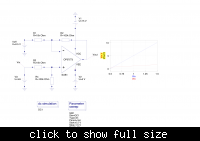# op amp offset and scaler question

Status
Not open for further replies.

#### steve1

##### Newbie level 4
Hello,

I have an input signal(0.5v to 1.5v) from a sensor and I want offset this to 0v- 10v using an opamp.

Vin min = 0.5v ; Vin max = 1.5v : Vout min = 0v : Vout max = 10v

Pls how will I do this? I got an equation on the web which states that my gain equals :

G = (Vout max - Vout min)/(Vin max - Vin min)
and my offset voltage will be :
Voffset = Vin max *|G|/(1 + |G|) and

Vout = G(Voffset - Vin) + Voffset

But my problem is that I dont no how the equation for Voffset was derived and its source. Also when I tried to use the above equations I got the following:

@ Vin = 0.5v Vout = 10v
@ Vin = 1.5 ; Vout = 0v

Pls this is reverse of what I want. I would like to have an Opamp cct that can really do the correct job.

You simply need a gain(Vin)=+10 (Vin @ the non-inverting input) and an offset=0.5V (@ the inverting input), s. the foll. figure:You can use an asymmetric power supply, but you need a negative power supply of a few volts to get the output down to 0V . And you need a stable Voff supply of 0.5V, perhaps via a poti, if your pos. pwr supply is stable enough. Its output impedance should be « R1 .
The generator (output) impedance of your input signal must be « (R3 + R4) .

V
Points: 2

Count component values and simulate.

View attachment 56531

Regards KAK

Thanks a lot. This is a real eye opener. It works

Status
Not open for further replies.